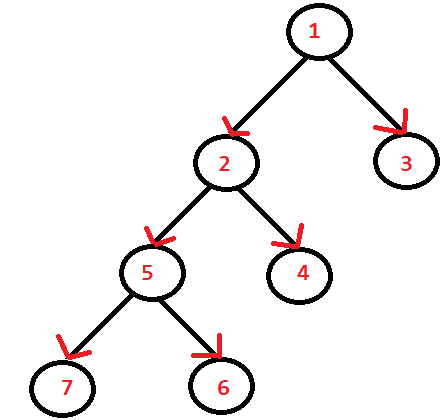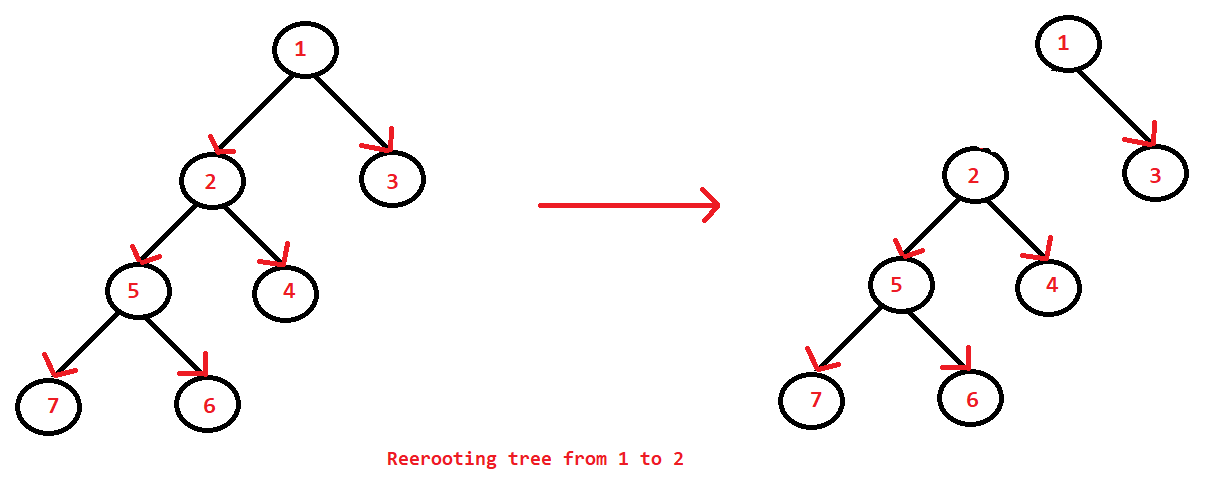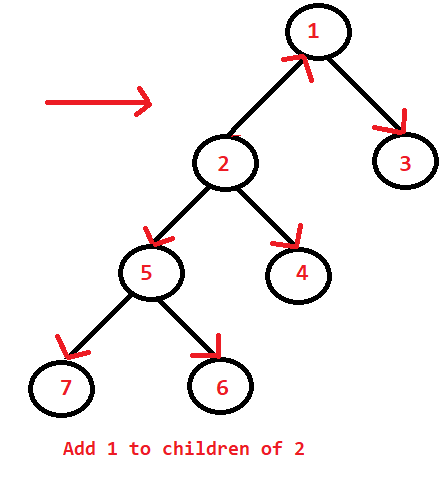# Sum of the Length of Paths from Every Node to All Other Nodes

## Introduction

In this problem, we will solve the most exciting topics of competitive programming, i.e., Trees and Dynamic Programming.

Both Trees and DP fulfill the void of the data structures and algorithms.

Dynamic Programming is used to solve optimization problems, while trees are the hierarchical data structure having nodes connected by edges.

## Problem Statement

Here we have to find the sum of the length of paths from it to all other nodes, using the Tree Rerooting technique in an undirected tree.

Before heading towards the approach, let's see what this problem looks like.

What do you mean by the Tree Rerooting technique?

Re-Rooting is a technique where we have to find a solution resulting from any chosen roots. We first see the solution to some fixed node and then start building the solution by rerooting the tree on all other nodes. It is a Dynamic Programming technique on trees.

Let us understand the problem with the help of an example.

We are provided with a tree having '7' nodes.

.Here arrows represent the path. The tree is undirected.

Output:

12 9 17 14 10 15 15

Explanation:

Node1: sum of the path for every node is 0(length of node 1)+ 1(length of node 2)+ 1(length of node 3)+ 2(length of node 5)+ 2(length of node 4)+ 3(length of node 7)+ 3(length of node 6) = 12.

Node2: sum of the path for every node is 0(length of node 2)+ 1(length of node 1)+ 2(length of node 3)+ 1(length of node 5)+ 1(length of node 4)+ 2(length of node 7)+ 2(length of node 6) = 9

Node3: sum of the path for every node is 0(length of node 3)+ 1(length of node 1)+ 2(length of node 2)+ 3(length of node 5)+ 3(length of node 4)+ 4(length of node 7)+ 4(length of node 6) = 17.

Node4: sum of the path for every node is 0(length of node 4)+ 1(length of node 2)+ 2(length of node 1)+ 3(length of node 3)+ 2(length of node 5)+ 3(length of node 7)+ 3(length of node 6) = 14.

Node5: sum of the path for every node is 0(length of node 5)+ 2(length of node 1)+ 3(length of node 3)+ 2(length of node 4)+ 1(length of node 2)+ 1(length of node 7)+ 1(length of node 6) = 10.

Node6: sum of the path for every node is 0(length of node 6)+ 1(length of node 5)+ 2(length of node 2)+ 3(length of node 4)+ 3(length of node 1)+ 4(length of node 3)+ 2(length of node 7) = 15.

Node7: sum of the path for every node is 0(length of node 7)+ 1(length of node 5)+ 2(length of node 6)+ 2(length of node 2)+ 3(length of node 4)+ 3(length of node 1)+ 4(length of node 3) = 15.

We will be solving this problem using two approaches in the Java language.

## Naive Approach

We will be using dynamic programming to compute the sum of length for every node to all other nodes.

Here, the dp[value] will store the sum of paths from a particular node for all subtrees.

The dp[value] will be calculated as follows:

dp[node] += dp[child] + size[child]

For each node, make the tree's root and find the dp from the above formula.

As the tree is rooted at 'node,' all the other nodes will be in its subtree. So dp[node] will be the required answer for 'node.'

### Implementation

import java.util.*;

class Solution
{

// Globally defining the variables
static int N = 7;
static int dp[] = new int[N];
static int total[] = new int[N];
static int len[] = new int[N];

// It will compute the total for every node of the subtree
//vector class implements dynamic array as well as it is synchronized
//in comparison to ArrayList.
static void dfs(int node, int parent, Vector<Integer> []tree)
{

// dp=0, as there is no paths
// size=1 as only one node is present initially
len[node] = 1;
dp[node] = 0;
for (int i : tree[node]) {

// For every neighbour of node
// which is not its parent
// 1. compute size and dp for
// i by dfs
// 2. update size and dp for node,
// based on i.
if (i != parent) {
dfs(i, node, tree);
len[node] += len[i];
dp[node] += dp[i] +
len[i];
}
}
}

// For creating the edge between the nodes
static void edge(int u, int v,
Vector<Integer> [] tree)
{

u--;
v--;

// Tree is undirected or we can say bidirectional
}

// To store the sum of paths in dp array.
static int[] pathSum(Vector<Integer> []tree)
{

// For root 'j'
// 1. compute dp for tree rooted at 'j'
// 2. as all nodes belong to some
// subtree of root, answer will be
// equal to dp
for (int j = 0; j < N; ++j) {
dfs(j, -1, tree);
total[j] = dp[j];
}
}

// Main function
public static void main(String[] args)
{

Vector<Integer> []tree = new Vector[N];
for (int i = 0; i < tree.length; i++)
tree[i] = new Vector<Integer>();
edge(1, 2, tree);
edge(1, 3, tree);
edge(2, 4, tree);
edge(2, 5, tree);
edge(5, 6, tree);
edge(5, 7, tree);
int[] ans = pathSum(tree);

for (int i = 0; i < N; ++i) {
System.out.print(ans[i]+ " ");
}
System.out.println();
}
}

Output

12 9 17 14 10 15 15

### Complexity Analysis

Time Complexity: Time complexity is O(N^2), where N is the number of nodes.

Space Complexity: O(N), due to dp array.

## Efficient Approach

The solution can be further optimized by calculating the answer for one root and rerooting the tree every time to calculate for other nodes. This is known as rerooting approach.

1) First we will run dfs for node 0 and calculate the DP values for this node where DP[i] will represent the sum of the distances of this node from all other nodes in its subtree.

2) Now, we will run another dfs in which we will calculate the answer for all the nodes using the DP values calculated in the 1st step.

3) For each node we will re-root the tree and make the current node to be the root and accordingly update the DP values using the below method:-

dp[node] += dp[child] + size[child].

Below is the pictorial representation of rerooting the nodes.Re-rooting is done from '1'to '2'.

• remove '2' from children of '1.'
• add '1' to children of '2.'

### Implementation

import java.util.*;

class Solution{

static int N = 7;
static int dp[] = new int[N];
static int total[] = new int[N];
static int len[] = new int[N];

// Dfs0 will compute the dp value
// for each node and will also find
// the size of each subtree
static void dfs0(int node, int parent, Vector<Integer> []tree)
{

// dp=0, as there is no paths
// size=1 as only one node is present initially
dp[node] = 0;
len[node] = 1;
for (int i : tree[node]) {

// For every neighbour of node
// which is not its parent
// 1. compute size and dp for
// i by dfs
// 2. update size and dp for node,
// based on i.
if (parent != i) {
dfs0(i, node, tree);
len[node] += len[i];
dp[node] += len[i] +
dp[i];
}
}
}

// Rerooting the tree from 'from' to 'to.'
static void rerooting (int from, int to)
{
// 'to' is no longer a child of 'from'
dp[from] -= len[to] + dp[to];
len[from] -= len[to];

// 'from' is now a child of 'to'
len[to] += len[from];
dp[to] += len[from] + dp[from];
}

static void dfs1(int node, int parent,Vector<Integer> []tree)
{
// The dfs1 considers 'node' as root
total[node] = dp[node];

// For all neighbours which are
// not parent of node
for (int i : tree[node]) {
if (parent != i) {
// Reroot the tree to 'i'
rerooting(node, i);

// Compute ans for 'i'
// as a root of tree with dfs
dfs1(i, node, tree);

// reroot the tree back
// to 'node'
rerooting(i, node);
}
}
}

// Creates a edge between u and v,
// given graph tree
static void edges(int u, int v,
Vector<Integer> [] tree)
{

u--;
v--;

// Tree is undirected or we can say bidirectional
}

// Function to calculate sum of paths
static int[] path(Vector<Integer> []tree)
{

// Compute answer for each subtree
dfs0(0, -1, tree);

// Compute answer for each node
// where each node is consider as root at
//the time of computation, rerooting
dfs1(0, -1, tree);
}

// Main Function
public static void main(String[] args)
{
int N = 7;
Vector<Integer> []tree = new Vector[N];
for (int i = 0; i < tree.length; i++)
tree[i] = new Vector<Integer>();

edges(1, 2, tree);
edges(1, 3, tree);
edges(2, 4, tree);
edges(2, 5, tree);
edges(5, 6, tree);
edges(5, 7, tree);
int[] ans = path(tree);

for (int i = 0; i < N; ++i) {
System.out.print(ans[i]+ " ");
}
System.out.println();
}
}

Output

12 9 17 14 10 15 15

### Complexity Analysis

Time Complexity: The time complexity is O(N), Where N is the number of nodes.

Space Complexity: O(N)

## FAQs

1. What do you mean by tree data structure?
The tree data structure consists of nodes connected with the help of the edges.

2. What do you understand by the tree rerooting technique?
Re-Rooting is a technique where we have to find a solution that can result from any chosen roots.
We can first calculate the size and answer for some fixed nodes and then calculate the answer by considering each node as the root.

## Key Takeaways

This blog discusses the various approaches to compute the sum of the length from every node to all other nodes. The two methods or ways can be summarized as follows:

• The first approach is a naive approach based on the transition in dynamic programming.

Transition :- for (child node):

dp[node] += dp[child] + size[child]

The time complexity taken by this approach is O(N^2).

• A second approach is efficient, using the rerooting technique and DP.

The time complexity taken by this approach is O(N).

Visit here to learn more about trees. And CodeStudio is a one-stop destination for various DSA questions typically asked in interviews to practice more such problems.

If you liked this blog, share it with your friends.

Happy Coding!!!